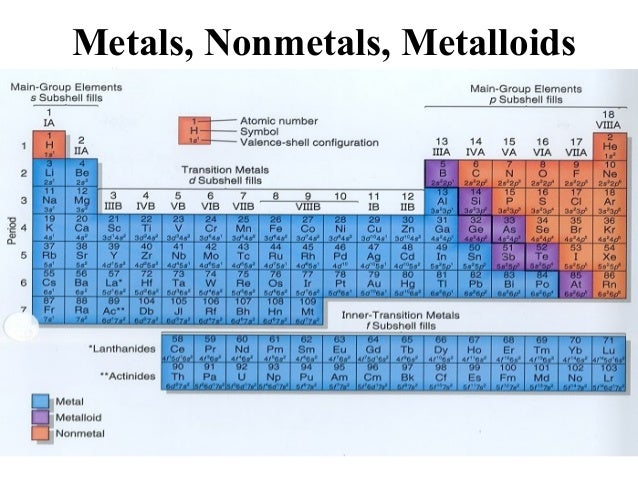# What is the name of the compound Ca(NO_3)_2?

May 7, 2016

Calcium nitrate

#### Explanation:

The name of the compound $C a {\left(N {O}_{3}\right)}_{2}$ is Calcium nitrate.

An ionic compound, is a compound that contains a metal element in its formula unit. Metal elements are found to the left of the stairs in a periodic table ( refer to the periodic table at the end of this answer ).

This is an ionic compound that is named in general as follows:

1. The cation (positive ion) which is represented by the metal is listed
first
2. the name of the metal stays the same (as you could see here, it is
Calcium)
3. The anion is listed second. In this case, it is a polyatomic anion which is the nitrate $N {O}_{3}^{-}$.

If the anion is a monatomic ion, for example $C {l}^{-}$, we would keep the root name and add ide at the end; the name is Chloride.

Example, $C a C {l}_{2}$ is called Calcium Chloride.

If the metal is a transition metal, we should add a roman number which represent its oxidation number.

Example, $C u {\left(N {O}_{3}\right)}_{2}$, it is called Copper(II) nitrate. Because copper is a transition metal and it has two oxidation numbers $+ 1$ and $+ 2$.

For example, $C u N {O}_{3}$ is called Copper(I) nitrate.

Of course, this is a big lesson to explain in few lines, but I thing this will help you to start learning how to name ionic compounds.$\textcolor{g r e y}{\text{Image source: http://www.slideshare.net/mae2388/parts-of-periodic-table}}$